The ABC metric

I have been involved recently in some discussions about the usefulness of the LOC (Lines Of Code) metric. As I explained in some previous posts, this is the first one I look at when doing an assessment of and application and its code quality. And I do it only to get an idea of the size of the application.

Then I will look at other metrics, for instance Cyclomatic Complexity (CC), to get an idea of its functional weight. It is also interesting to cross-check these metrics, for instance: average number of LOC by java class or method, or average CC by class or method. This helps to spot classes or methods that are difficult to understand, either because a developer will have to go up and down into a large file to understand the flow of instructions, either because there are a lot of conditions or loops which make the flow complex to understand. Thus the risk of creating defects is higher when a change is implemented.

When you talk about LOC in a discussion, you will probably have some people telling you that this metric should not be used to measure productivity or effort, which I do agree that it’s incorrect. It should be limited to measure size.

Function Points (FP) is often considered as the best metric to measure productivity. It sure has some advantages, especially that it can be used on specifications, before starting development, so it helps to measure the effort needed to implement specs into code and to estimate the man/days needed. However, this metric has, in my opinion, a main inconvenience: it is not easy to automate and a FP expert will be very cautious, not to say suspicious, if he had to use function points calculated by a code analysis tool.

While working on previous posts with the City Model plugin for Sonar, I discovered that eXcentia, the Spanish company at the origin of this plugin, also has created one to calculate an ABC metric.

There is a good description from Jerry Fitzpatrick, the author of this metric, that you can find here.

This metric is based on:

• A: Assignment of a value to a variable.
• B: Branch (call) to a function or a new object.
• C: Condition, a test.

Then, I created a class ‘TestAssignment.java’ composed of 50 assignments like:

int var1=0;
int var2=0;

I launched a Sonar analysis from Jenkins (if you want to know how to do it, just have a look at previous posts like this one).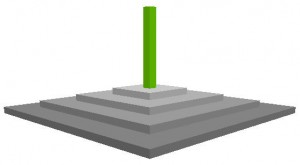Here is the result: an unique building for an unique class. And here are the values that I get:

• ABC metric = 50, corresponding to the statements we have created.
• LOC = 55, corresponding to the 50 assignments and some few lines to declare the class and an unique method.
• Complexity = 1, as there is absolutely no complexity.Then, I created a new class ‘TestCondition.java’ with the following code:

public class TestCondition {
int var1=0;
public TestCondition() {
if (this.var1 = 0){
this.var1 = 1;
}
else{
this.var1 = 2;
}

and the previous test duplicated 8 times, to get approximatively the same number of lines of code than the first class TestAssignment. Note that I also have 25 assignments: 3 in each test x 8 duplications of the test = 24 + 1 corresponding to the initial declaration of the variable var1.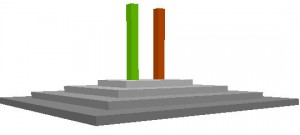Now I have two buildings on the same size as these two classes have (almost) the same number of LOC but one is red because its level of complexity is more important.

But the ABC metric is just slightly above the 25 assignments in the code so the complexity does not weight so much on the result.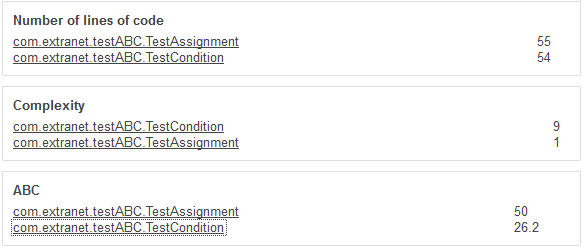As you can imagine, the third class I created was ‘TestBranch.java’ with 50 ‘new’ statements:

TestCondition myCondition1 = new TestCondition();
TestCondition myCondition2 = new TestCondition();
TestCondition myCondition50 = new TestCondition();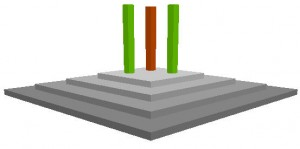The heigth is still the same but now the ABC value is the most important as we have 50 assignments with the 50 branch statements.

However, it is not the double of the value for the TestAssignement class. This one has 50 assignments and an ABC value of 50, while the TestBranch class has also 50 assignments AND 50 branchs, but an ABC value slightly above 70.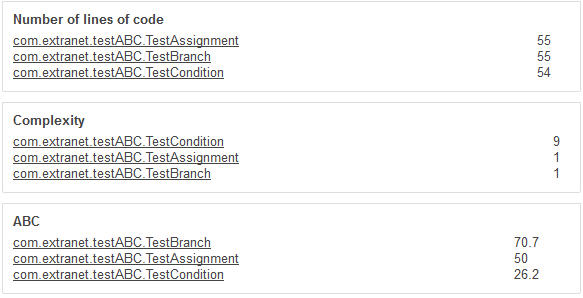First, we can see different values for three classes with the same LOC. So, the ABC metric is independant of the number of lines of code.

Now, I am not sure that it can be used as a measure of productivity or effort as we can see that the A of the assignments weights very much in its calculation. Conditions are clearly undervalued. It is obvious that the effort to introduce a change into a structure of 8 ‘if-else’ is higher than into a sequence of 50 assignments. Especially that some of these conditions will often be nested.

And most of the times, a branch will create new assignments. And we could see that adding 50 branchs to 50 assignments does not double the result when compared to a code with 50 assignments without any branch. This is due to the formula of calculation of the ABC metric. When in fact, I think that the effort for the programmer will be more than multiplied by two.

So, which use can we do of the ABC metric? Because of the predominance of the A of Assignments and the undervaluation of the C of Conditions and, in a minor way, of the B of Branchs, I believe it is more oriented to data than to logic. Which is not a problem, as we already have the Cyclomatic Complexity metric to estimate functional complexity.

So I did the following, define a new model of City with:

• Heigth still based on LOC.
• Width based on the A of Assignments of the ABC metric.
• The color based on the overall value of the metric, from 1 (green) to 100 (red).

Here is what I get now for the three classes of my test: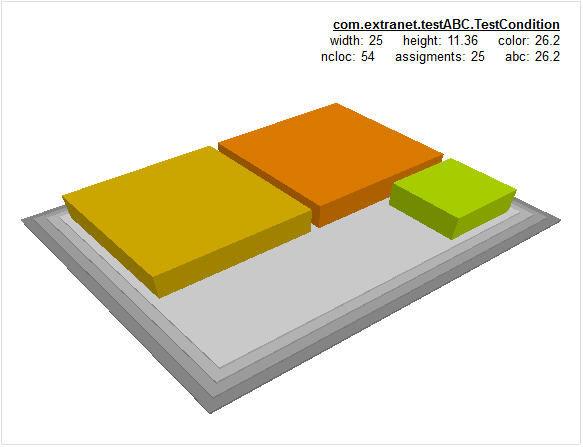The three buildings still have the same heigth.

The small green one is the TestCondition class with a width of 25 corresponding to its 25 assignments and a color corresponding to its ABC value of 26.2, thus undervaluing the C of Conditions.

The two other blocks corresponding to the TestAssignment and the TestBranch classes have the same width of 50 corresponding to their number of assignments but the TestBranch building is going more on the red than the TestAssignment building, because of the added Branch statements.

This visual representation helps to understand how to use the ABC metric. Again, I would not use it to measure effort but a certain kind of ‘data size’ if I take in account the width of each building, and an overall measure of how risky is a component, based on the color of the building. Risky because it either has some complexity or more probably some coupling added to the ‘data weight’.

The ABC plugin from eXcentia comes with its one widget (we have seen in our last post how to add it to our personalized Sonar dashboard):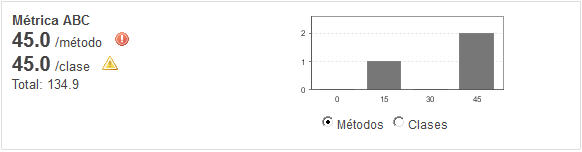It shows us:

• A total of 134.9 for the whole application.
• An average of 45 for classes, which represents a moderate risk.
• An average of 45 for methods, which represents an high risk and that some refactoring should be done for the 2 methods that are over an ABC value of 45.

So this widget is in line with our use of the ABC metric, based on the risk to introduce a defect when implementing a change in a component.

Now if I want to get a better estimation of this risk, the ABC plugin allows me to do some drill-down.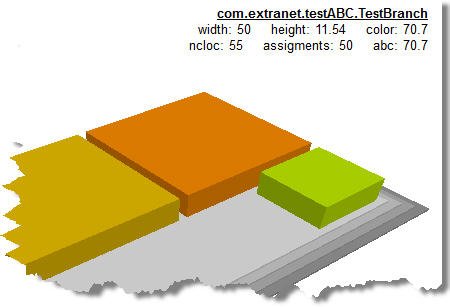Here I just selected the ‘riskiest building’ in the City representation corresponding to the class TestBranch, with its different values.

I just have to click on its name to drill-down to a description of the ABC measures for this class, with the details of the A, B, C values used to calculate the metric and the average values for method and classes.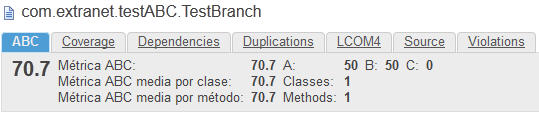The mix of the two eXcentia plugins for Sonar, City Model and ABC metric provides another visual evaluation of the level of risk and the necesity of refactoring an application.

Here is a real example: I have been adding to my analisis a package that I know has some big classes:You can see on the right the ‘TestABC’ district with the three classes we have created for our tests. Now, in this City representation, I changed the expression to calculate the width of the building based on the average Assignments per method (instead of the total for the class previously).

In the figure above, I am pointing here to the high red tower on the left, a ‘SesionUsuario’ class that manages all the data for an user’s session in the application. We can see:

• More than 2 700 LOC, which explains the height of the tower.
• An overall ABC value of 538.6, which explains the red color.
• That this value is mostly based on 522 Assignments.
• An high number of methods: 67, which explains the width of the building (522 Assignments / 67 methods).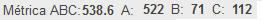A drill-down to this class shows that there are 71 Branchs and 112 Conditions in its code. It confirms the overvaluation of the Assignments into the overall ABC value.

Now, with the initial parameters of the City Model, this class would appear as high, with a bigger width based on its number of methods, but completely green because the CC by method would be low. So that would be a big component but not necessarily high in the refactoring list.

Using the ABC values, the width is much lower, as the ABC per method is low, but the color is completely red, which immediately attracts the attention on the level of risk to introduce a change into this class. So now, this component is high in our refactoring list.

There would be other factors to take in account, as the overall quality of the code based on programming malpractices. What we can say here is that:

• LOC is a measure of size, not suitable to evaluate functional weight or productivity or effort.
• CC is a good measure of functional weight based on the number of logic paths but not necessarily a good indicator of refactoring priorities.
• I believe the ABC metric is a good measure of ‘data weight’ based on the overvaluation of the assignments in its formula, and thus a good complement to CC.
• The City modelization we have built, based on the eXcentia plugins for Sonar, helps us to evaluate the risky components and to quickly point to the primary candidates to a refactoring list.

It is likely that we could find a lot of different City representations to address different questions. I will have fun to experiment that for future posts.

This entry was posted in Sonar 360 on .